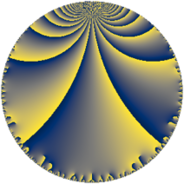# Properties

 Label 49.6.eLevel $49$ Weight $6$ Character orbit 49.e Rep. character $\chi_{49}(8,\cdot)$ Character field $\Q(\zeta_{7})$ Dimension $138$ Newform subspaces $1$ Sturm bound $28$ Trace bound $0$

# Related objects

## Defining parameters

 Level: $$N$$ $$=$$ $$49 = 7^{2}$$ Weight: $$k$$ $$=$$ $$6$$ Character orbit: $$[\chi]$$ $$=$$ 49.e (of order $$7$$ and degree $$6$$) Character conductor: $$\operatorname{cond}(\chi)$$ $$=$$ $$49$$ Character field: $$\Q(\zeta_{7})$$ Newform subspaces: $$1$$ Sturm bound: $$28$$ Trace bound: $$0$$

## Dimensions

The following table gives the dimensions of various subspaces of $$M_{6}(49, [\chi])$$.

Total New Old
Modular forms 150 150 0
Cusp forms 138 138 0
Eisenstein series 12 12 0

## Trace form

 $$138 q - 5 q^{2} + 13 q^{3} - 389 q^{4} + 67 q^{5} + 331 q^{6} - 56 q^{7} + 305 q^{8} - 918 q^{9} + O(q^{10})$$ $$138 q - 5 q^{2} + 13 q^{3} - 389 q^{4} + 67 q^{5} + 331 q^{6} - 56 q^{7} + 305 q^{8} - 918 q^{9} - 771 q^{10} + 410 q^{11} - 1316 q^{12} + 483 q^{13} + 2597 q^{14} + 2557 q^{15} - 7357 q^{16} - 1422 q^{17} - 2480 q^{18} - 6758 q^{19} - 3647 q^{20} - 399 q^{21} - 857 q^{22} - 2392 q^{23} - 3571 q^{24} - 8066 q^{25} - 343 q^{26} + 16396 q^{27} + 32102 q^{28} + 30341 q^{29} + 13716 q^{30} - 32712 q^{31} - 26993 q^{32} - 16775 q^{33} + 35163 q^{34} - 12019 q^{35} + 35461 q^{36} - 34872 q^{37} + 13383 q^{38} - 20671 q^{39} + 165169 q^{40} + 51233 q^{41} - 13741 q^{42} - 17203 q^{43} - 4267 q^{44} + 30777 q^{45} - 74611 q^{46} + 64264 q^{47} - 247084 q^{48} - 70350 q^{49} - 263036 q^{50} - 57453 q^{51} - 36043 q^{52} + 124590 q^{53} + 144840 q^{54} + 90977 q^{55} + 404404 q^{56} - 103899 q^{57} + 24861 q^{58} - 8079 q^{59} + 72065 q^{60} - 236659 q^{61} - 173227 q^{62} - 119805 q^{63} + 105381 q^{64} - 33383 q^{65} + 536577 q^{66} + 19716 q^{67} - 256340 q^{68} - 202795 q^{69} + 194803 q^{70} + 61778 q^{71} - 392108 q^{72} - 157760 q^{73} - 328319 q^{74} + 355167 q^{75} - 61334 q^{76} - 24402 q^{77} + 497217 q^{78} - 73876 q^{79} - 1340760 q^{80} - 815116 q^{81} + 248976 q^{82} + 303107 q^{83} + 1250354 q^{84} + 205487 q^{85} + 1083219 q^{86} + 197027 q^{87} + 1052915 q^{88} + 219783 q^{89} + 82170 q^{90} - 837235 q^{91} + 35936 q^{92} + 138365 q^{93} - 571908 q^{94} - 610016 q^{95} - 1054494 q^{96} + 762020 q^{97} - 2160767 q^{98} - 1090772 q^{99} + O(q^{100})$$

## Decomposition of $$S_{6}^{\mathrm{new}}(49, [\chi])$$ into newform subspaces

Label Dim $A$ Field CM Traces $q$-expansion
$a_{2}$ $a_{3}$ $a_{5}$ $a_{7}$
49.6.e.a $138$ $7.859$ None $$-5$$ $$13$$ $$67$$ $$-56$$